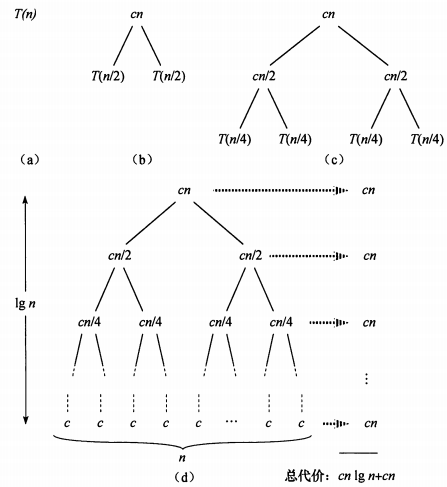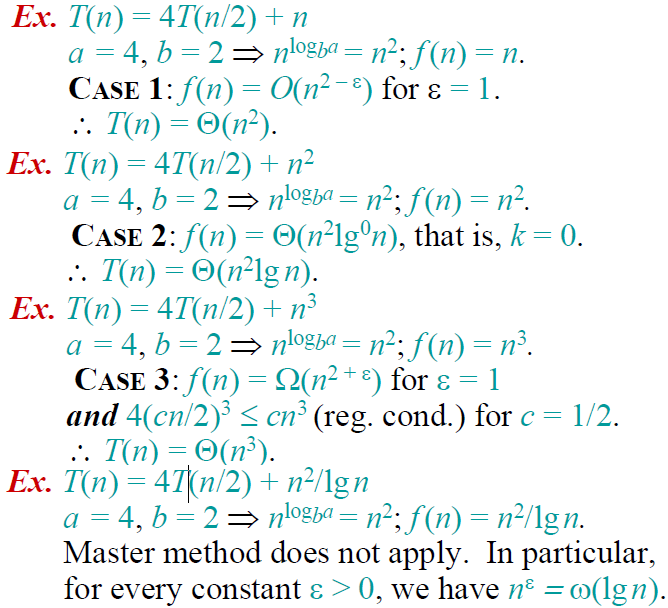# 算法导论------递归算法的时间复杂度求解

## 1.算法设计与分析概述

在总结递归算法的时间复杂度分析之前，应该明确几组概念。
算法仅仅是求解问题的解决方案，这个解决方案本身并不是问题的答案，而是能获得答案的指令序列。只有通过执行算法才可以获得求解问题的答案。
从算法是否递归调用的角度看，算法可以分为非递归算法和递归算法。
非递归算法时间复杂度分析较为简单，通常是计算算法中基本语句执行次数，一般都是一个关于问题规模n的表达式，然后用渐近符号 ΘOoΩω $Θ、Ο、o、Ω、ω$表示出算法的时间复杂度。
递归算法是采用分治的方法，把一个“大问题”分解出若干个相似的“小问题”求解。在分析算法复杂度时，关键是根据递归过程建立递推关系式，然后求解递推关系式，得到算法执行的时间表达式（一般都与问题规模n相关），最后用渐近符号 ΘOoΩω $Θ、Ο、o、Ω、ω$表示出算法的时间复杂度。
在《算法导论》、《算法设计与分析》这2门课中，我们已经学习一些通用的算法设计技术，如增量法、分治法、贪心法、动态规划、线性规划、回溯法、分支限界法等；在算法设计完成后，对算法的复杂度进行分析是必然的，所以本篇的中心将围绕算法时间复杂度展开。

## 2.非递归算法分析

void fun(int n){
int x=0;
for(int i=1;i<=n;i++){
for(int j=1;j<=i;j++){
for(int k=1;k<=j;k++){
x++;             //基本语句
}
}
}
}

f(n)=i=1nj=1ik=1j1=i=1nj=1ij=i=1ni(i+1)2=....=O(n3) $f(n)=\sum\limits_{i=1}^n\sum\limits_{j=1}^{i}\sum\limits_{k=1}^{j}1=\sum\limits_{i=1}^n\sum\limits_{j=1}^{i}j=\sum\limits_{i=1}^n\frac{i(i+1)}{2}=....=O(n^3)$，时间复杂度为 O(n3) $O(n^3)$

1. 等差数列 {ak} $\left\{a_k\right\}$求和： k=1nak=n(a1+an)2 $\sum\limits_{k=1}^na_k=\frac{n(a_1+a_n)}{2}$
2. 等比数列 {aqk} $\left\{aq^k\right\}$求和： k=0naqk=a(1qn+1)1q $\sum\limits_{k=0}^naq^k=\frac{a(1-q^{n+1})}{1-q}$
3. 调和级数 {1k} $\left\{ \frac{1}{k} \right\}$求和： k=1n1k=lnn+O(1) $\sum\limits_{k=1}^n\frac{1}{k}=lnn+O(1)$（需要用微积分知识证明）
4. 对数级数 lg1+lg2+...+lgn=lg(n!)=Θ(nlgn) $\lg 1+\lg 2+...+\lg n=\lg(n!)=Θ(n\lg n)$（利用Stirling公式证明）
5. 放缩1：用序列中的最大项代替序列中的每个项，这种方法可以表示为： k=1naknamax $\sum\limits_{k=1}^na_k≤na_{max}$
6. 放缩2：在等比数列中，假设存在常数 r<1 $r<1$，使得 ak+1akr $\frac{a_{k+1}}{a_k}≤r$对一切 k0 $k≥0$成立，那么有：
k=0nakk=0a0rk=a0k=0rk=a01r
7. 放缩3：对每个b>1和每个a>0，都有 logbn=o(na) $\log_bn=o(n^a)$
8. 1+3+5+...+(2n1)=n2 $1+3+5+...+(2n-1)=n^2$
9. 12+22+32+...+n2=16n(n+1)(2n+1)=O(n3) $1^2+2^2+3^2+...+n^2=\frac{1}{6}n(n+1)(2n+1)=O(n^3)$（幂方级数，上限比幂次高出一阶）
10. 13+23+33+...n3=[12n(n+1)]2=O(n4) $1^3+2^3+3^3+...n^3=\left[ \frac{1}{2} n(n+1)\right]^2=O(n^4)$
11. 各种收敛级数，不再一 一列举。

## 3.递归算法分析

### 3.1利用数列知识

1. 累加法：递推关系式为 an+1an=f(n) $a_{n+1}-a_{n}=f(n)$采用累加法。
2. 累乘法：递推关系式为 an+1an=f(n) $\frac{a_{n+1}}{a_{n}}=f(n)$采用累乘法。
3. 构造法：递推关系式为(1) aa+1=pan+q $a_{a+1}=pa_n+q$，(2) aa+1=pan+qn $a_{a+1}=pa_n+q^n$，都可以通过恒等变形，构造出等差或等比数列，利用等差或等比数列的定义进行解题，其中的构造方法可通过待定系数法来进行。
4. 和化项法：递推公式为 Sn=f(n) $S_n=f(n)$ Sn=f(an) $S_n=f(a_n)$一般利用
an={S1SnSn1n=1n>=2
5. 用特征方程求解递推方程（感觉比较生僻，不做解释）
6. 迭代法： 从原始递推方程开始，反复将对于递推方程左边的函数用右边的等式代入，直到得到初值，然后将所得的结果进行化简。
例如在调用归并排序mergeSort(a,0,n-1)对数组 a[0...n1] $a[0...n-1]$排序时，执行时间 T(n) $T(n)$的递推关系式为：
T(n)={O(1)2T(n2)+O(n)n=1n>=2

其中， O(n) $O(n)$为merge()所需要的时间，设为 cn $cn$（c为正常量）。因此：
T(n)=2T(n2)+cn=2(2T(n4)+cn2)+cn=22T(n4)+2cn=23T(n8)+3cn=...=2kT(n2k)+kcn=nO(1)+cnlog2n=O(nlog2n),(n=2k,k=log2n)

忽略求解细节。在我们求解递归式时，因为最终是要求得一个时间上限，所以在求解时常常省略一些细节。比如mergeSort(a,0,n-1)运行时间的实际递归式应该是：

T(n)=O(1)T(n2)+T(n2)+O(n)n=1n>=2

类似的，我们也可以用迭代法求解汉诺塔递归求解时的时间复杂度。但遗憾的是，迭代法一般适用于一阶的递推方程。对于二阶及以上（即 T(n) $T(n)依赖它前面更多个递归项$）的递推方程，迭代法将导致迭代后的项太多，从而使得求和公式过于复杂，因此需要将递推方程化简，利用差消法等技巧将高阶递推方程化为一阶递推方程。如在求快速排序算法平均时间复杂度 T(n) $T(n)$的递推方程， T(n) $T(n)$依赖 T(n1)T(n2)...T(1) $T(n-1)、T(n-2)、...、T(1)$等所有的项，这样的递推方程也称为全部历史递推方程。（这里省略快速排序算法平均复杂度T(n)的求解过程）

### 3.2代入法

1. 猜测解的形式；
2. 用数学归纳法求出解中的常数，并证明解是正确的。

遗憾的是并不存在通用的方法来猜测递归式的正确解，需要凭借经验，偶尔还需要创造力。即使猜出了递归式解的渐近界，也有可能在数学归纳证明时莫名其妙的失败。正是由于该方法技术细节较为难掌握，因此这个方法不适合用来求解递归方程，反而比较适合作为其他方法检验手段。在此不做总结。可以翻阅《算法导论》进行学习。

### 3.3递归树

递归树是一棵结点带权值的树。初始的递归树只有一个结点，它的权标记为 T(n) $T(n)$；然后按照递归树的迭代规则不断进行迭代，每迭代一次递归树就增加一层，直到树中不再含有权值为函数的结点（即叶结点都为 T(1) $T(1)$）。下面以递归方程

T(n)={O(1)2T(n2)+O(n)n=1n>=2;(n=2k,k=log2n)

• 第一步： 把根结点 T(n) $T(n)$用根是 cn $cn$、左结点为 T(n2) $T(\frac{n}{2})$、右结点为 T(n2) $T(\frac{n}{2})$的子树代替（即：以分解、合并子问题需要的代价为根，分解得到的子问题为叶的子树。其中常量c代表求解规模为1的问题所需的时间）；（如下如 (a)(b) $(a)\rightarrow(b)$
• 第二步：把叶结点按照“第一步”的方式展开； T(n2) $T(\frac{n}{2})$用根是 cn/2 $cn/2$、左节点为 T(n4) $T(\frac{n}{4})$、右结点为 T(n4) $T(\frac{n}{4})$的子树代替。（如下如 (b)(c) $(b)\rightarrow(c)$
• 第三步：反复按照“第一步”的方式迭代，每迭代一次递归树就增加一层，直到树中不再含有权值为函数的结点（即叶结点都为 T(1) $T(1)$）。（如下如 (c)(d) $(c)\rightarrow(d)$在得到递归树后，将树中每层中的代价求和，得到每层代价，然后将所有层的代价求和，得到所有层次的递归调用的总代价。在上图(d)部分中，完全展开的递归树高度为 lgn $\lg n$(树高为根结点到叶结点最长简单路径上边的数目)，所有递归树具有 lgn+1 $\lg n+1$层，所以总代价为 cn(lgn+1) $cn*(\lg n+1)$，所有时间复杂度为 Θ(nlgn) $Θ(n\lg n)$

总结：递归树模型求解递归方程，本质上就是迭代思想的应用，利用递归方程迭代展开过程构造对应的递归树，然后把每层的时间代价进行求和。不过递归树模型更直观，同时递归树也克服了二阶及更高阶递推方程不方便迭代展开的痛点。

### 3.4主方法求解递推式

主方法为如下形式的递归式提供了一种“菜谱”式的求解方法，如下所示

T(n)=aT(n/b)+f(n)

T(n)=aT(n/b)+f(n)

1. 若对某个常数 ε>0 $ε>0$ f(n)=O(n(logba)ε) $f(n)=O(n^{(\log_b{a})-ε})$，则 T(n)=Θ(nlogba) $T(n)=Θ(n^{\log_ba})$
2. 若 f(n)=Θ(nlogba) $f(n)=Θ(n^{\log_b{a}})$，则 T(n)=Θ(nlogbalgn) $T(n)=Θ(n^{\log_ba}\lg n)$
3. 若对某个常数 ε>0 $ε>0$ f(n)=Ω(n(logba)+ε) $f(n)=Ω(n^{(\log_b{a})+ε})$，且对某个常数 c<1 $c<1$和所有足够大的n有 af(n/b)cf(n) $af(n/b)≤cf(n)$，则 T(n)=Θ(f(n)) $T(n)=Θ(f(n))$

在使用主定理之前，要比较 f(n)(nlogba) $f(n)和(n^{\log_b{a}})$的大小，这个大小不是算术意义上的大小比较，而是要在多项式意义上比较。以上三种情况在多项式意义上并未覆盖 f(n) $f(n)$的所有可能性。情况1和情况2之间有一定间隙；情况2和情况3之间也有一定间隙。如果f(n)落在这两个间隙中，或者情况3中 正则条件不成立，就不能使用主方法来求递归式。
如在递归式： T(n)=2T(n/2)+nlgn $T(n)=2T(n/2)+n\lg n$中，因为 nlogba=n<f(n)=nlgn $n^{\log_b{a}}=n，但是 f(n) $f(n)$并不大于n一个多项式因子 nε $n^ε$ ,因为对于给定的 ε>0 $ε>0$当n足够大时，均有 nε>lgn $n^ε>\lg n$。所以找不到这样 ε>0 $ε>0$，该递归式落入了情况2和情况3之间的间隙，不能使用主定理。
最后给出主定理应用的几个练习题：## 4.参考资料

1. 《算法导论》第三版
2. 《算法设计与分析》屈婉玲著，清华大学出版社
3. 《算法设计与分析》李春葆著，清华大学出版社

09-175万+12-092150
07-011137
10-013万+
01-225648
10-282843
02-17413
06-082594
07-293140
12-07267
06-17
03-202万+
12-081万+
11-082万+
08-231298
06-121075
03-22955
05-19260
09-271993